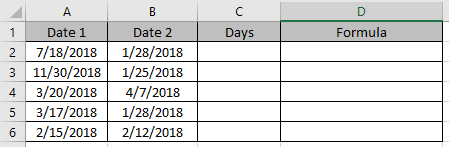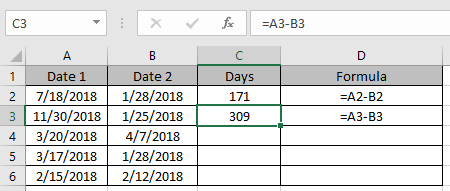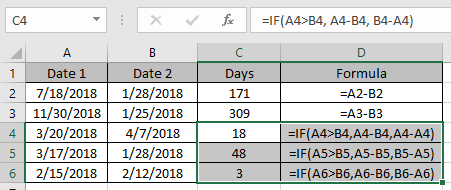# How to Get days between dates in Excel

Excel stores dates as numbers, a simple mathematical formulation explained. So just need to subtract the two dates it will get the days between the two dates.

=later_date - earlier date

Or

=IF(date1>date2, date1 - date2, date2 - date1)

Use the below formula, if you don’t know which date to put first.
Let’s understand this function using it an example.Use the formula in C2 and D2 cells.

=A2-B2This formula gets you negative results if the date1 < date2.
If you don’t want to care which is smaller, just use the below formula in remaining cells

=IF(A4>B4, A4-B4, B4-A4)Use the above function formulas to get the days between dates in Excel

Hope you understood how to get the days between dates in Excel. Explore more articles on Excel Date & TIME functions here. Please feel free to state your query or feedback for the above article.

Related Articles:

How to use the DAYS function in Excel

How to Count Days in Month in Excel

How to Calculate days,months and years in Excel

How to Calculate days, years and months from certain date in Excel

Popular Articles

50 Excel Shortcut to Increase Your Productivity : Get faster at your task. These 50 shortcuts will make you work even faster on Excel.

How to use the VLOOKUP Function in Excel : This is one of the most used and popular functions of excel that is used to lookup value from different ranges and sheets.

How to use the COUNTIF function in Excel : Count values with conditions using this amazing function. You don't need to filter your data to count specific values. Countif function is essential to prepare your dashboard.

How to use the SUMIF Function in Excel : This is another dashboard essential function. This helps you sum up values on specific conditions.

Terms and Conditions of use

The applications/code on this site are distributed as is and without warranties or liability. In no event shall the owner of the copyrights, or the authors of the applications/code be liable for any loss of profit, any problems or any damage resulting from the use or evaluation of the applications/code.